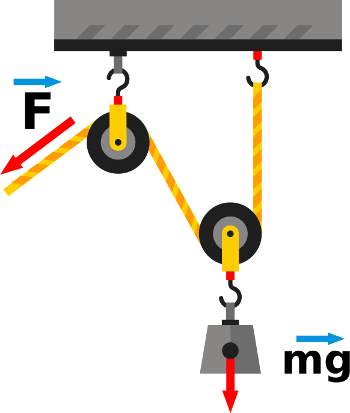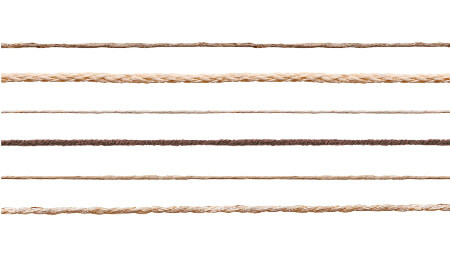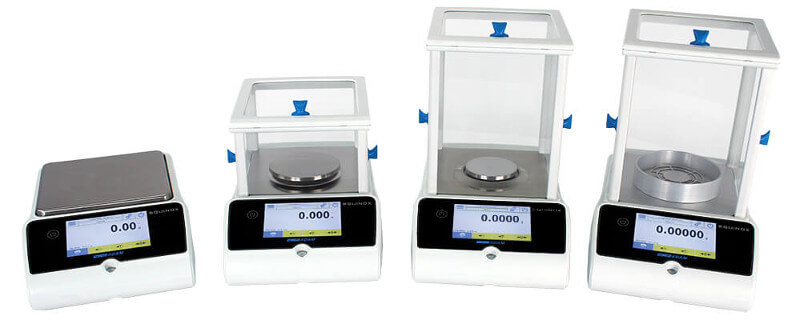• Good morning
• 29/06/2022

# The difference between mass and weight

Release: 1st August 2019

Adam Equipment, Stratlab's 'go-to' supplier of balances have published the following articles (May, 2019), used here with permission.

# The Differences between Mass and WeightMass and weight seem to be interchangeable whenever they’re discussed, yet they’re anything but. So what is mass? What is weight? How do they differ and why is it important to know the difference? How are they linked? We’ll answer these questions and more in this blog post. Please keep in mind this explanation is simplified.

## What is mass?

Mass is a measure of how much matter an object is composed of. Usually, mass is measured in grams or kilograms. Here’s a fun fact: in the imperial system, mass is not measured in ounces or pounds, but with a unit called the slug. Mass is linked to Force, as it can be measured by determining the amount of Force it takes to change the direction or speed of an object. You’re probably familiar with this equation: F (force) = m (mass) times a (acceleration). Mass is also linked to gravity, which is basically the attraction between two objects that have mass depending on distance. However, they are not related; gravity has no effect on mass.Mass is also indestructible (like energy, and it’s a scalar as well), and can never be equal to zero (think about it: mass is the measure how much of matter is in an object. How can an object be composed of zero matter?).

## What is weight?

Here’s something you might not have known: the metric unit for weight is the Newton, not the gram. The imperial unit for weight is the pound. Remember mass and gravity being linked? Weight is the gravitational interaction between objects that have mass. If you’ve looked at the differences between mass and weight before, you’ve probably seen something about your mass on Earth and the Moon being the same, but you’ll weigh less on the Moon than you do on Earth because the Moon’s mass is not the same as Earth’s (so its gravity is not the same). Weight can be equal to zero (depending on the gravity) and is force (and therefore a vector).## Why the Confusion?

Well, for one, mass and weight are not only related, but proportional. If you know something’s mass, you can find out its weight, and vice versa, using the formula W (weight) = m (mass) times g (gravity, on Earth usually valued at 9.8 N/kg). Since we live on Earth and usually don’t have to consider mass on another planet (unless you’re a scientist), we usually use these terms somewhat interchangeably. Furthermore, weight being expressed in Newtons would be confusing to most people. If I say my cat weighs 10 pounds (or 4.5 kilos), you know he’s about average, but if I say he’s about 44 Newtons, that’s meaningless to most people.

## Scales, Balances, Mass and Weight

Scales give you the weight of something – to be technical, they measure how much force is exerted on the load cell (weight is measured in Newtons, remember?) - and then uses gravity to give you your mass. Other scales (like TBBs) measure mass by comparing the known mass and the unknown mass.## Why it’s Important not to Confuse them

Mass is a key variable in a lot of different formulas for basic concepts. It must be exact, or else it can throw everything into disarray. Gravity and even altitude can affect weight, and even the slightest miscalculation could be disastrous or completely wrong in fields like aerospace, astronomy, physics, chemistry and more. Because mass is a fundamental property, it shapes our understanding of the world and how it works, and how we can affect it.

# How to Use the Textile Function on your BalanceOur new Equinox series of lab balances feature a textile function. But what does that mean? What are the applications? How are balances involved in textiles? We’ll answer these questions and more in this blog post. Don’t forget our manuals are all available for free to download on our website if you’re not sure how to use a feature on your balance.

## What is the textile function?

The textile function is designed to determine the number of yarns in a bundle. You measure a bundle of yarn to get a yarn count.

## What is yarn?

Yarn can be defined as “a long, continuous length of interlocked fibers, suitable for use in the production of textiles, sewing, crocheting, knitting, weaving, embroidery and rope making.” When you take a bundle of yarn, you can see that the fibers are interlocked and form a thicker thread. You can unwind and separate them.## Units of measurements

There are two systems of measurements for measuring yarn, each with their own units. We’ll focus on the units offered by the Equinox to keep things simple.

In the Direct System (weight / length), the yarn count is expressed as the number of mass units per length units. It follows that the higher the count, the coarser and thicker the yarn. While the units may change, the formula to calculate yarn count remains the same: Yarn Count = (Sample Weight / Length Unit) / (Sample Length / Weight Unit) (or N = (W/l) / (L/w)). All the count systems under the direct system use a fixed length and a variable weight.

Tex (Tt):
The yarn count is the weight in grams in 1000m (1km) of yarn.
Denier (Td):
The yarn count is the weight in grams in 9000m (9km) of yarn.

The direct system is usually used to measure textiles such as polyester, nylon, silk, acrylic, and various synthetic textiles.In the Indirect System, the yarn count is expressed at the length per mass unit. It follows that the higher the count, the finer and thinner the yarn. The formula for the Indirect System is the following: Yarn Count = (Sample Length / Weight Unit) / (Sample Weight / Length Unit) (or N = (L/w) / (W/l)). All the count systems under the indirect system use a variable length and a fixed weight.

Metric (Nm):
The number of hanks (1 hank = 480 yard of yarn) per kilogram.
English Cotton Count (Nc in the Equinox, but also abbreviated Ne or NeC):
The number of hanks (1 hank = 480 yard of yarn) per pound.

The indirect system is often used to measure cotton.

## Why does the amount of yarn in a bundle matter?

Yarn count is a unit that, according to the Textile Institute, “indicates the mass per unit length or the length per unit mass of yarn.” Essentially, it’s a numerical way to express how fine, coarse, thick or thin yarn is (it’s related to the thread count of sheets; the finer the yarn, the higher the thread count). It’s a way to standardize measuring in the textile industry and to make evaluation easier.

Yarn count can also express the durability of the fabric weaved from the yarn and its applications. A thick yarn is better suited to items such as towels or durable work uniforms because it’s unlikely to rip easily, whereas fabric from thin yarn is smooth and soft, but can tear easily. Despite common belief that the higher the yarn/thread count, the better the fabric, textiles with a very high yarn count can be fragile and difficult to wash, so higher is not always better.

## Why use a balance to measure yarn count?

There are manual ways to calculate yarn count, but they can be clunky and time consuming.  Yarn count is also a standard in the industry and it’s less likely to need conversion than other ways of measuring it. Using a balance is quicker and easier, and if there is a high volume of fabric to be evaluated, it’s less prone to errors. No one wants to rely on hand calculations when creating an industrial batch of fabric.  A balance provides an efficient way to check the quality and applications of a yarn and is an important part of the process during material research and development.## How does the balance calculate yarn count?

When you use the textile function on the Equinox, you pick your measuring unit, and fill out the data you have. For example, if you used Tex, you would fill out the known length of your yarn in meters or yards. The balance weighs the sample and uses these numbers to calculate the yarn count using the unit’s system.

## Equinox balances and textiles

The Equinox features a color touchscreen with menus that are easy to navigate. The menu prompts are intuitive and you can create a database of frequently-used samples to speed up repetitive tasks, so you don’t have to reenter the same information over and over again. You can also perform a weighing statistics through the database. The results can be printed and stored in a USB drive. You can preset a tare or enter a custom one during measurement.

Eight analytical Equinox models offer capacities from 120g to 510g and 0.1mg readability. Twelve precision models offer capacities from 360g to 8200g and readabilities of 1mg and 0.01g.